Question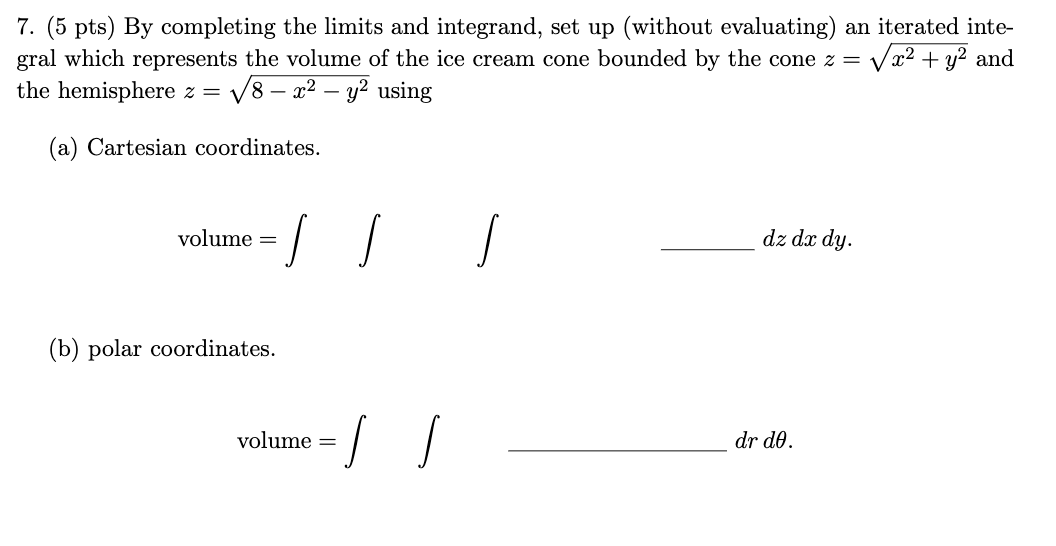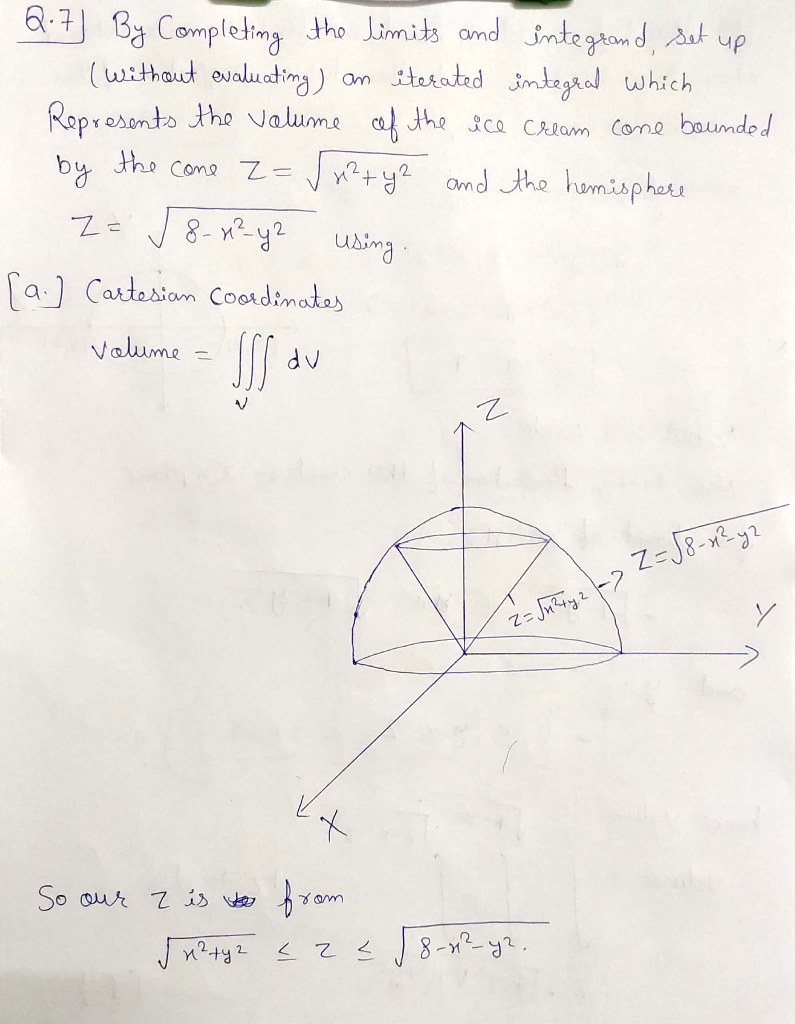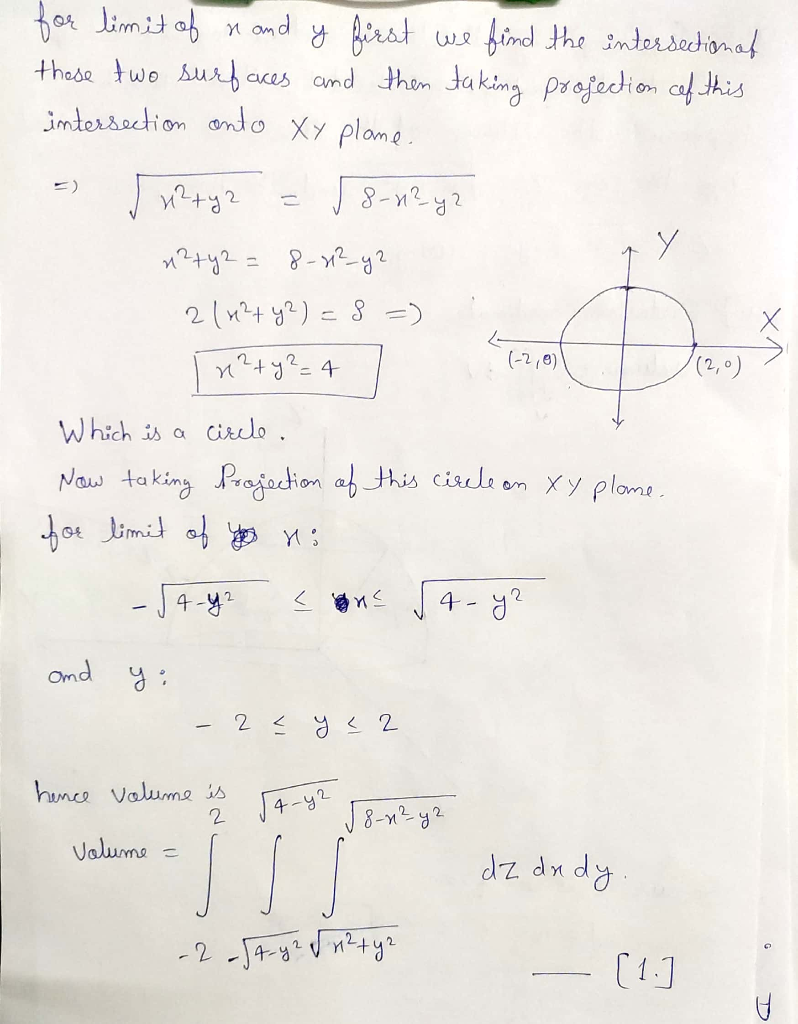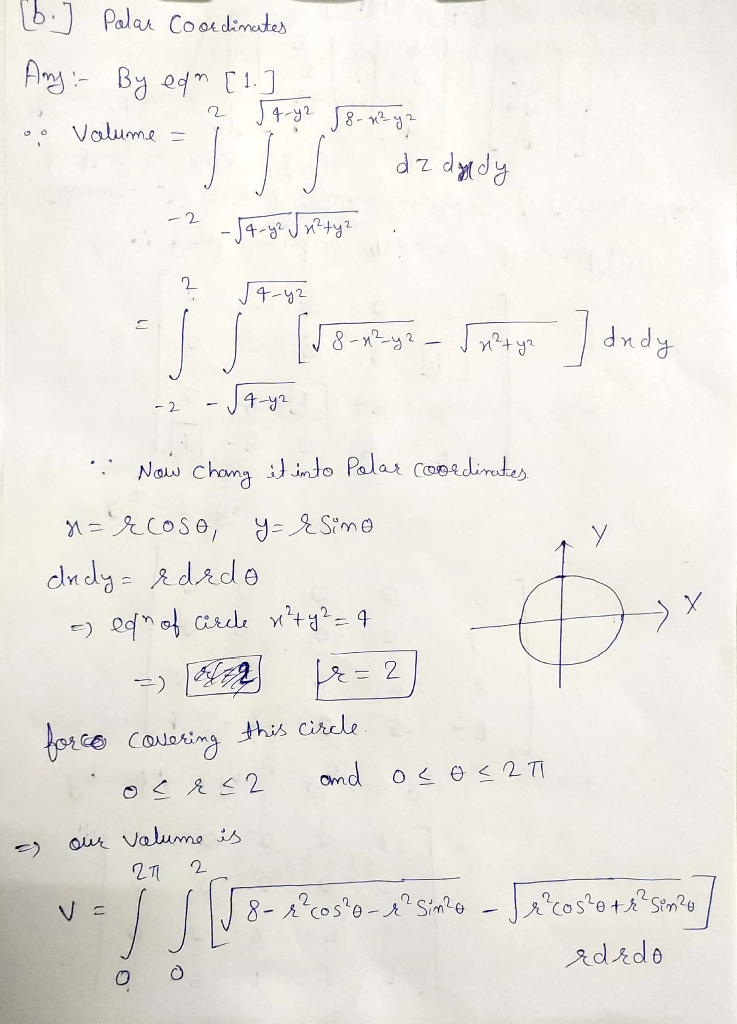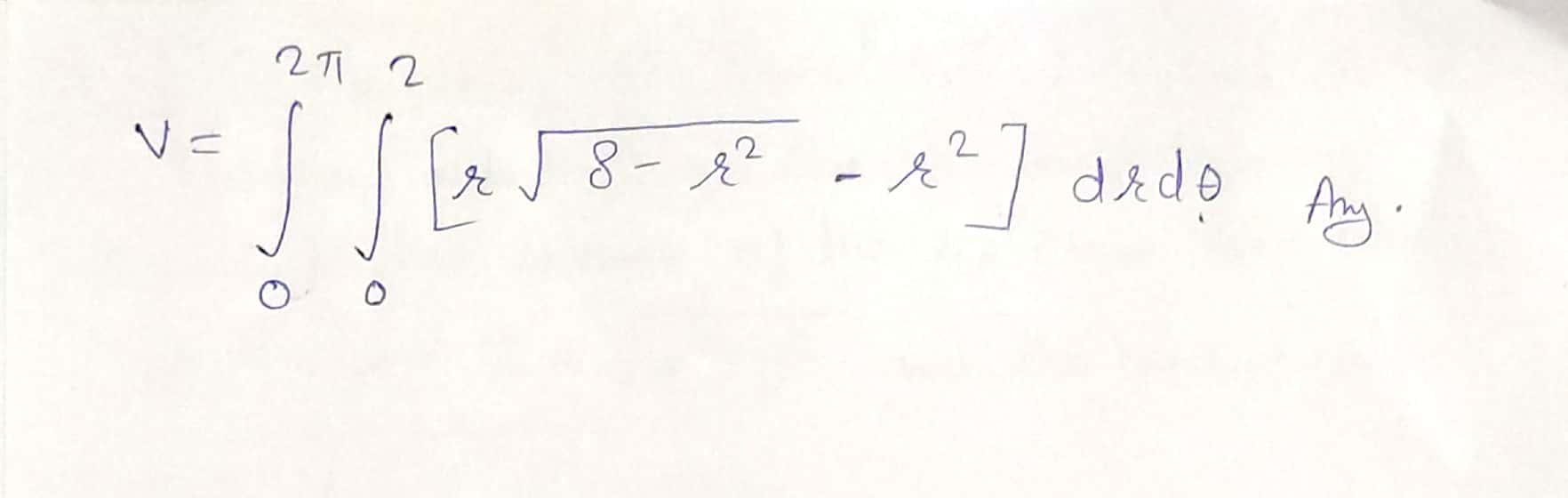#### Earn Coins

Coins can be redeemed for fabulous gifts.

Similar Homework Help Questions
• ### 7. (5 pts) By completing the limits and integrand, set up (without evaluating) an iterated inte-...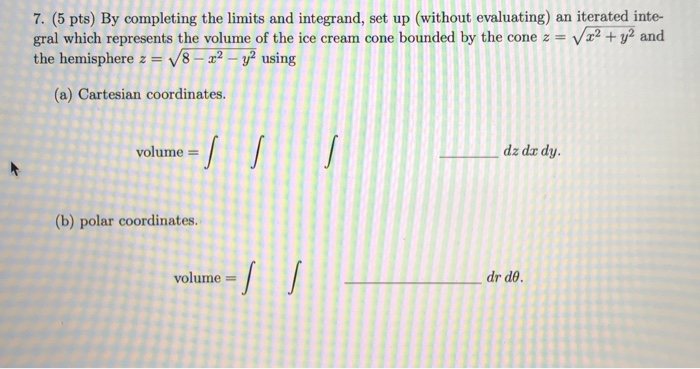7. (5 pts) By completing the limits and integrand, set up (without evaluating) an iterated inte- gral which represents the volume of the ice cream cone bounded by the cone z = V x2 + y2 and the hemisphere z = V8 - 22 - y2 using (a) Cartesian coordinates. volume = dz dar dy. (b) polar coordinates. volume = dr de.

• ### 7. (5 pts) By completing the limits and integrand, set up (without evaluating) an iterated inte-gral...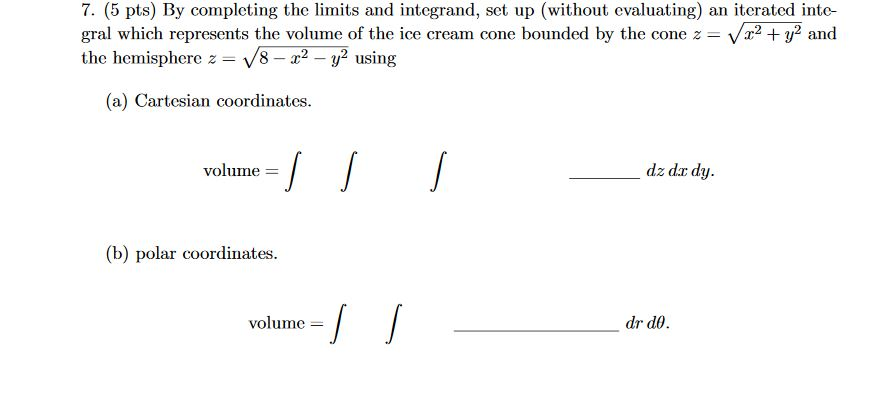7. (5 pts) By completing the limits and integrand, set up (without evaluating) an iterated inte-gral which represents the volume of the ice cream cone bounded by the cone z=√x2+y2andthe hemisphere z=√8−x2−y2using(a) Cartesian coordinates. 7. (5 pts) By completing the limits and integrand, set up (without evaluating) an iterated inte- gral which represents the volume of the ice cream cone bounded by the cone z = Vr2 + y2 and the hemisphere z = 18 - 22 - y2 using...

• ### 6. (4 pts) Consider the double integral∫R(x2+y)dA=∫10∫y−y(x2+y)dxdy+∫√21∫√2−y2−√2−y2(x2+y)dxdy.(a) Sketch the region of integration R in Figure 3.(b)...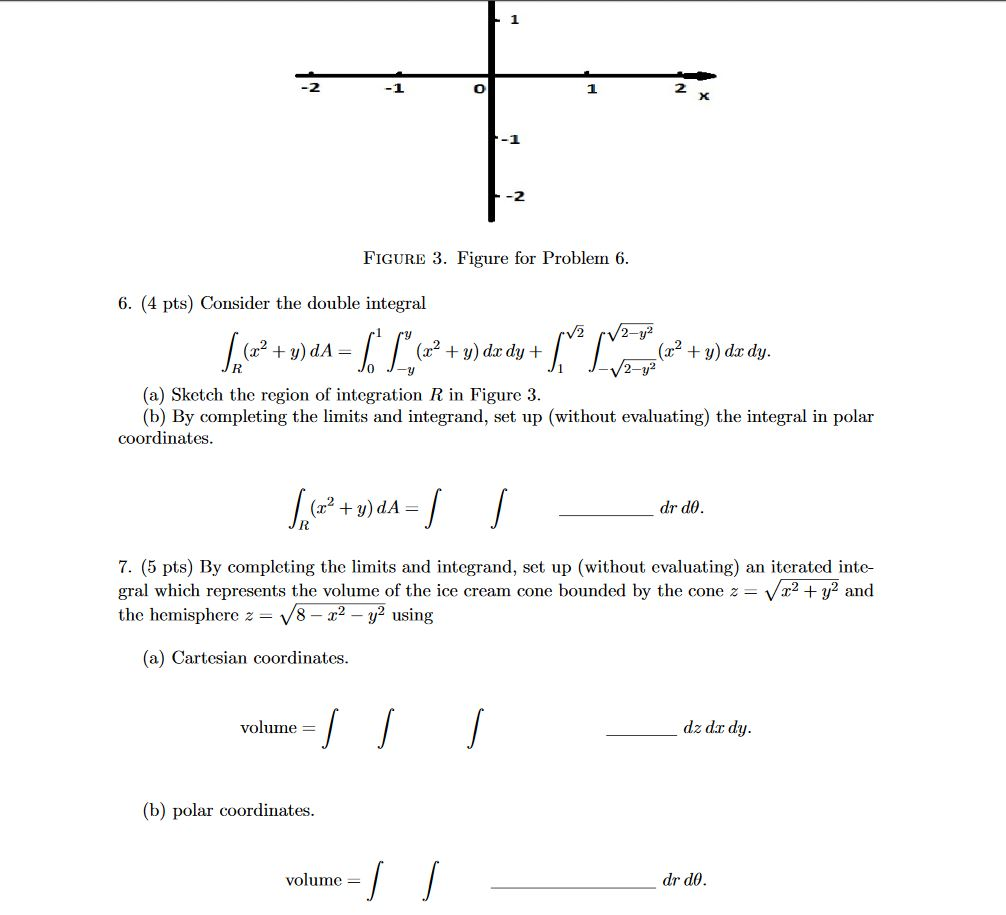6. (4 pts) Consider the double integral∫R(x2+y)dA=∫10∫y−y(x2+y)dxdy+∫√21∫√2−y2−√2−y2(x2+y)dxdy.(a) Sketch the region of integration R in Figure 3.(b) By completing the limits and integrand, set up (without evaluating) the integral in polar coordinates.∫R(x2+y)dA=∫∫drdθ.7. (5 pts) By completing the limits and integrand, set up (without evaluating) an iterated inte-gral which represents the volume of the ice cream cone bounded by the cone z=√x2+y2andthe hemisphere z=√8−x2−y2using(a) Cartesian coordinates.volume =∫∫∫dz dxdy.(b) polar coordinates.volume =∫∫drdθ. -1 -2 FIGURE 3. Figure for Problem 6. 6. (4 pts)...

• ### 6. (4 pts) Consider the double integral∫R(x2+y)dA=∫10∫y−y(x2+y)dxdy+∫√21∫√2−y2−√2−y2(x2+y)dxdy.(a) Sketch the region of integrationRin Figure 3.(b) By completing...6. (4 pts) Consider the double integral∫R(x2+y)dA=∫10∫y−y(x2+y)dxdy+∫√21∫√2−y2−√2−y2(x2+y)dxdy.(a) Sketch the region of integrationRin Figure 3.(b) By completing the limits and integrand, set up (without evaluating) the integral in polar coordinates. -1 -2 FIGURE 3. Figure for Problem 6. 6. (4 pts) Consider the double integral V2 /2-y² + = (x2 + y) dx dy + + y) do dy. 2-y2 (a) Sketch the region of integration R in Figure 3. (b) By completing the limits and integrand, set up (without evaluating)...

• ### Can anyone help me figure out the limits and what the function would be? Thank you!...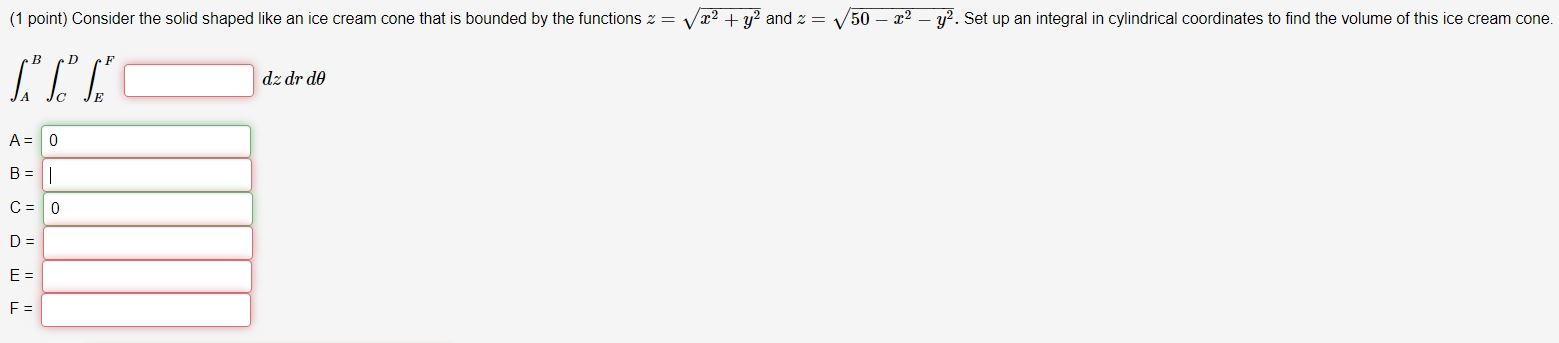Can anyone help me figure out the limits and what the function would be? Thank you! (1 point) Consider the solid shaped like an ice cream cone that is bounded by the functions 2= v x2 + y2 and 2 = 50 - 22 - y2. Set up an integral in cylindrical coordinates to find the volume of this ice cream cone. B D F "T" dz dr de A = 0 B = - C = 0 D =...

• ### 6. (4 pts) Consider the double integral∫R(x2+y)dA=∫10∫y−y(x2+y)dxdy+∫√21∫√2−y2−√2−y2(x2+y)dxdy.(a) Sketch the region of integration R in Figure 3.(b)...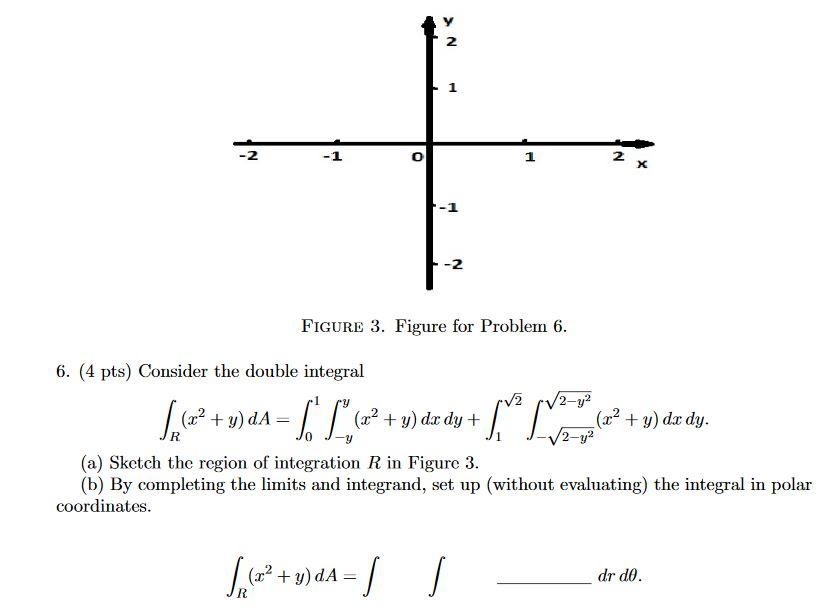6. (4 pts) Consider the double integral∫R(x2+y)dA=∫10∫y−y(x2+y)dxdy+∫√21∫√2−y2−√2−y2(x2+y)dxdy.(a) Sketch the region of integration R in Figure 3.(b) By completing the limits and integrand, set up (without evaluating) the integral in polar coordinates. 2 1 2 X -2 FIGURE 3. Figure for Problem 6. 6. (4 pts) Consider the double integral V2 2-y2 (2? + y) dA= (32 + y) dx dy + (x2 + y) dx dy. 2-y? (a) ketch the region of integration R in Figure 3. (b) By completing...

• ### Enter the correct limits of integration. Use increasing limits of integration. Set up the iterated integral...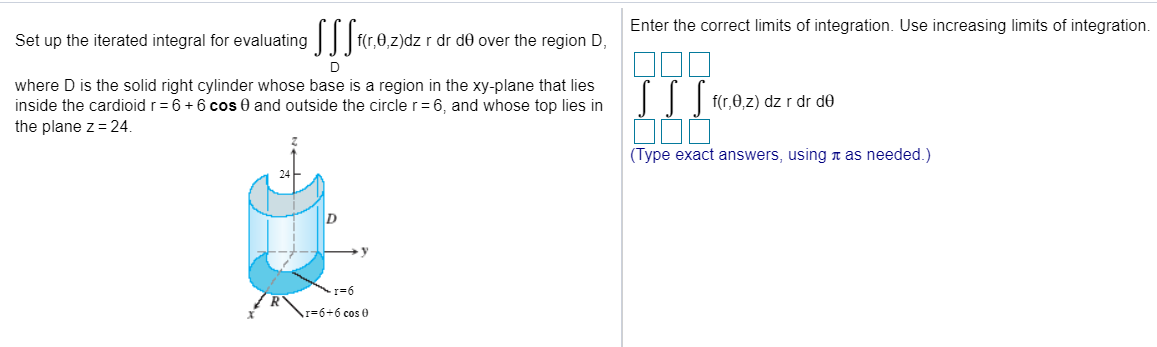Enter the correct limits of integration. Use increasing limits of integration. Set up the iterated integral for evaluating SS S40,0,.2)dz f(r,0,z)dz r dr de over the region D, D where D is the solid right cylinder whose base is a region in the xy-plane that lies inside the cardioid r = 6 +6 cos 0 and outside the circle r=6, and whose top lies in the plane z = 24 SSS fr, 0z) dz r dr de (Type exact answers,...

• ### 1 -1 O 1 2 x FIGURE 3. Figure for Problem 6. 6. (4 pts) Consider...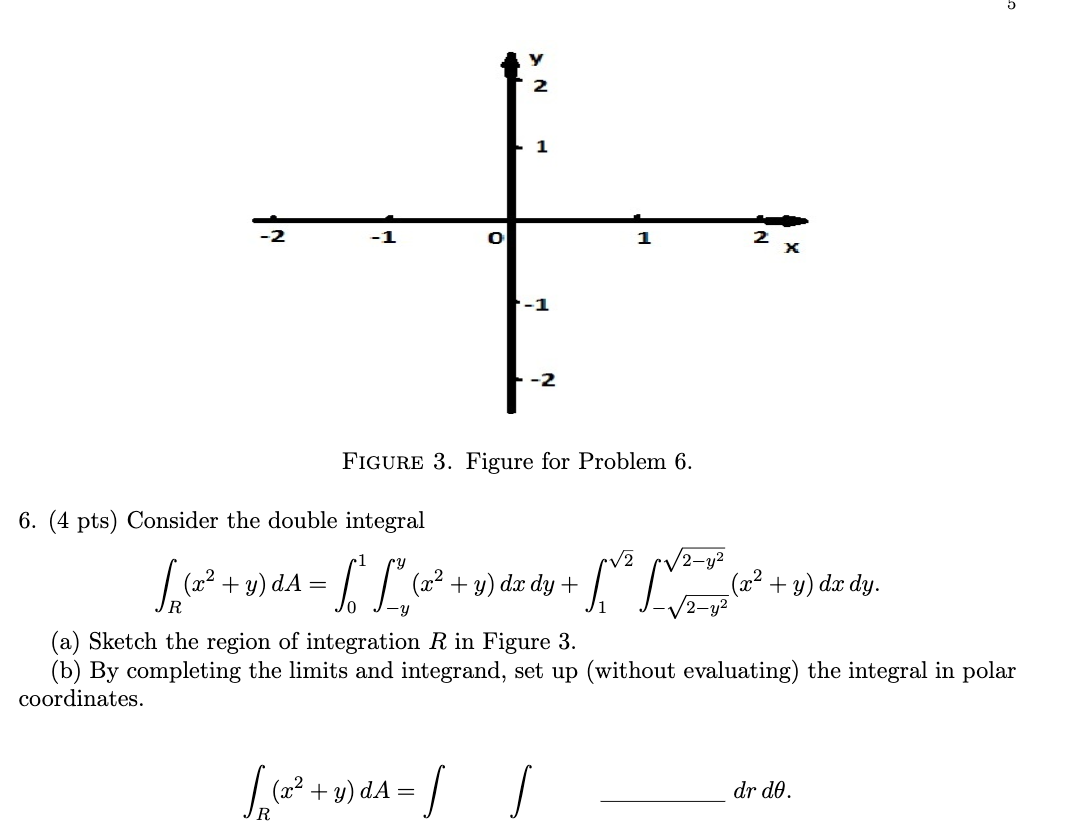1 -1 O 1 2 x FIGURE 3. Figure for Problem 6. 6. (4 pts) Consider the double integral 2 Spa (22 + y)da = [ L. (x2 + y) dx dy + √2-y² (x2 + y) dx dy. (a) Sketch the region of integration R in Figure 3. (b) By completing the limits and integrand, set up (without evaluating) the integral in polar coordinates. Sep (+2 +y)dA = dr do.

• ### 16. Question Details LarCalc11 14.6.017. (3865000) Set up a triple integral for the volume of the...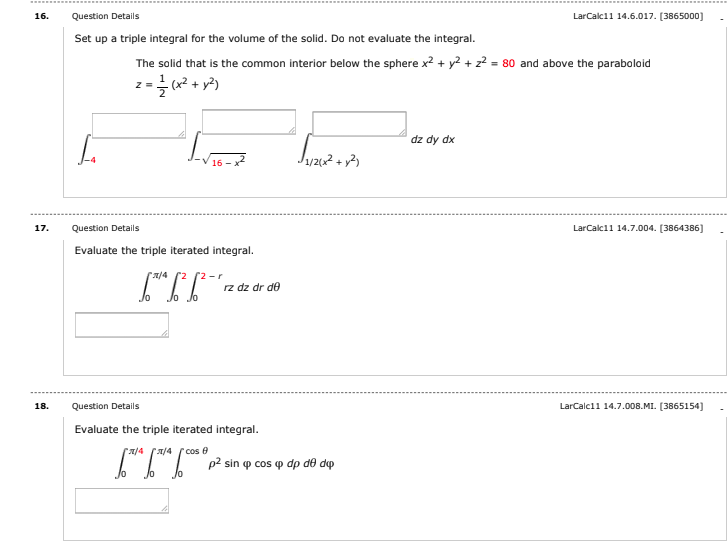16. Question Details LarCalc11 14.6.017. (3865000) Set up a triple integral for the volume of the solid. Do not evaluate the integral. The solid that is the common interior below the sphere x2 + y2 + 2+ = 80 and above the paraboloid z = {(x2 + y2) dz dy dx L J1/2012 + y2 Super 17. LarCalc11 14.7.004. (3864386] Question Details Evaluate the triple iterated integral. 6**6*6*2 2/4 2 2r rz dz dr de Jo lo 18. Question Details...

• ### Question Use cylindrical coordinates to set up the triple integral needed to find the volume of...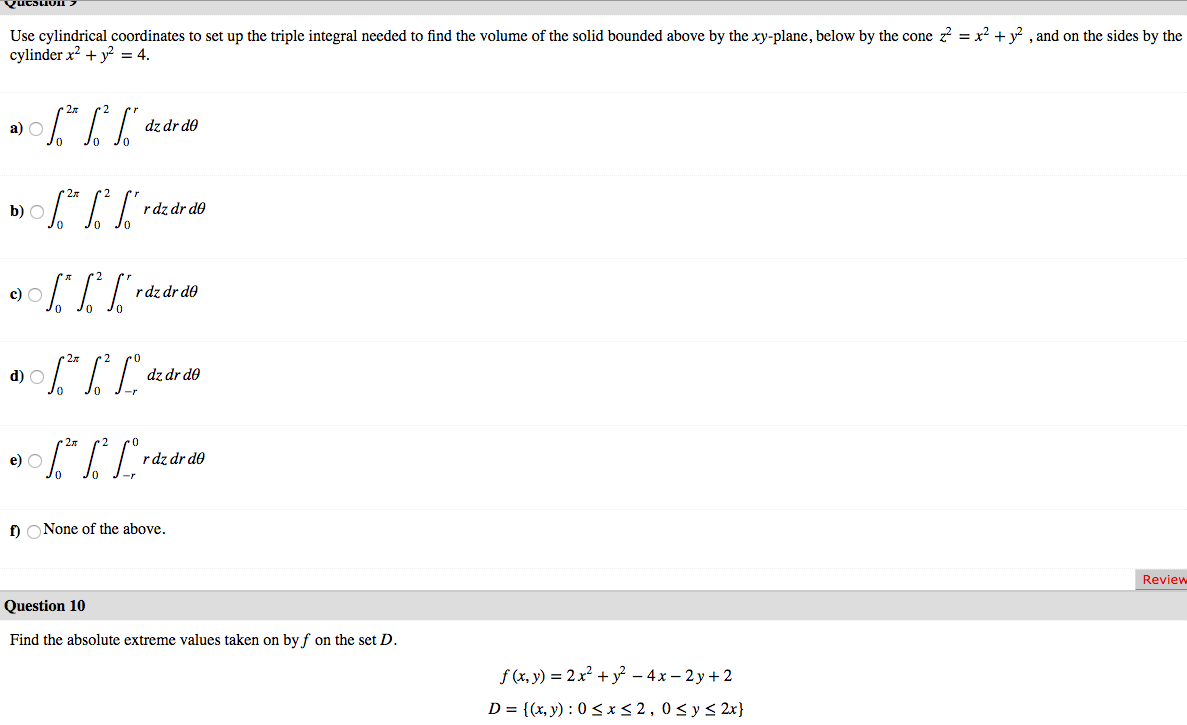Question Use cylindrical coordinates to set up the triple integral needed to find the volume of the solid bounded above by the xy-plane, below by the cone z = x2 + y2 , and on the sides by the cylinder x2 + y2 = 4. a) 06.* %* ["dz dr do b) \$* * S*rde de do JO 0% ] raz dr do a) \$** [Lºdz dr do 0906.*|*Lºrdz dr do 2 po dz dr do Jo J- O J-...